# RS Aggarwal Solutions for Class 6 Chapter 8 Algebraic Expressions Exercise 8C

RS Aggarwal Solutions for Class 6 Chapter 8 Exercise 8C are available in PDF format for free download. Subtraction of Algebraic Expressions is the topic that is covered under this exercise. Students can refer to the solutions to get a better understanding of the concept. These solutions provide accurate answers which are easily understood by the students. Practicing problems using RS Aggarwal solutions increase the capacity of answering at a good speed. Download PDF from their respective links.

## Download PDF of RS Aggarwal Solutions for Class 6 Chapter 8 Algebraic Expressions Exercise 8C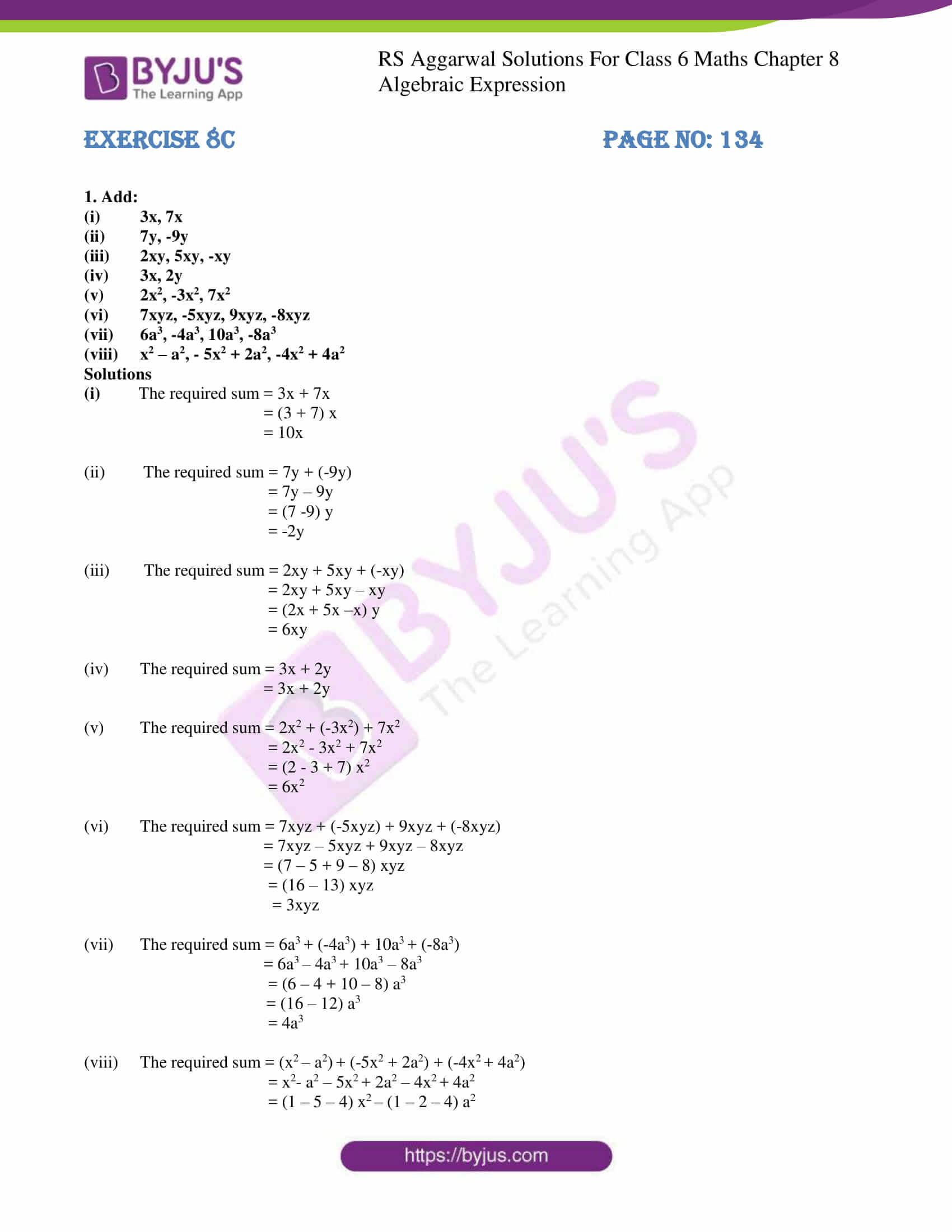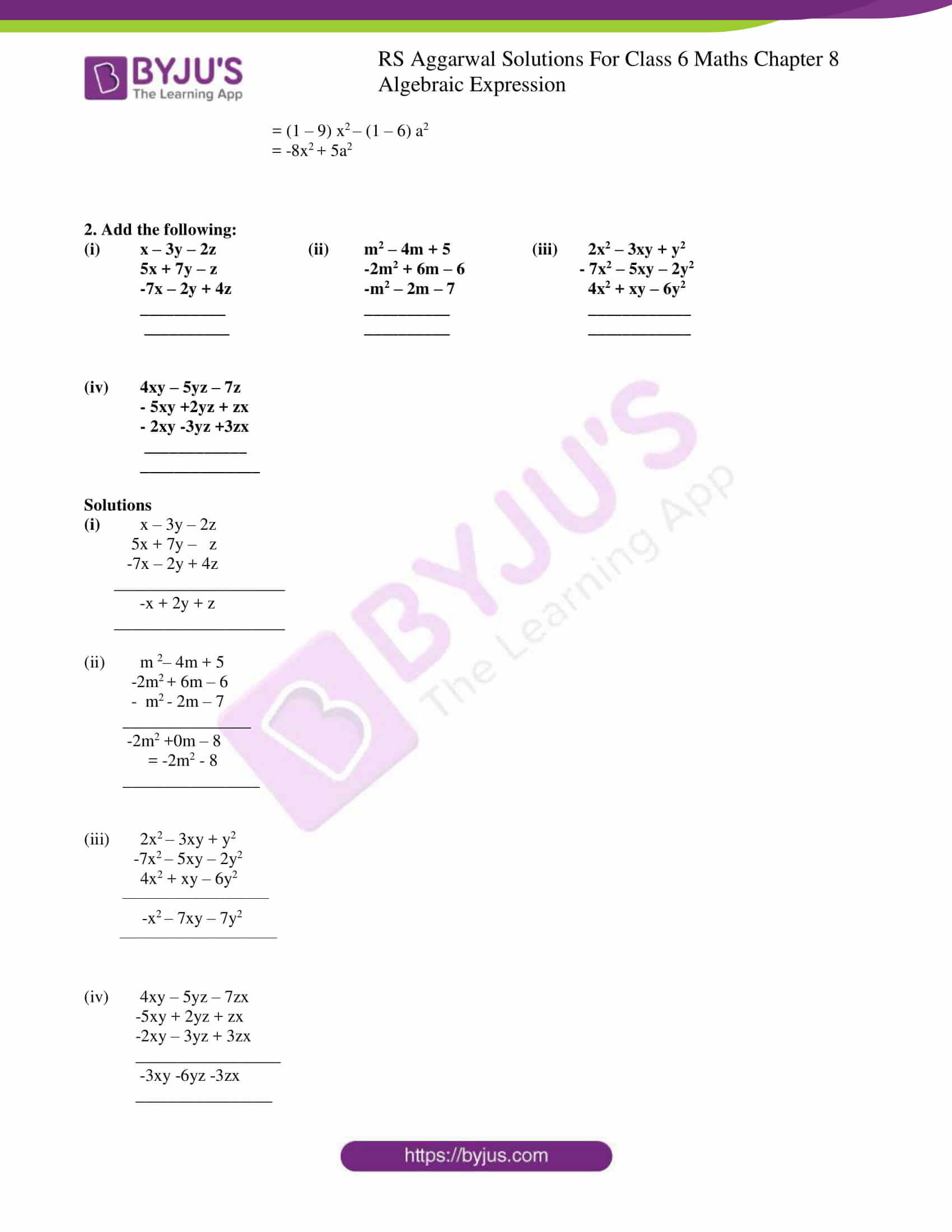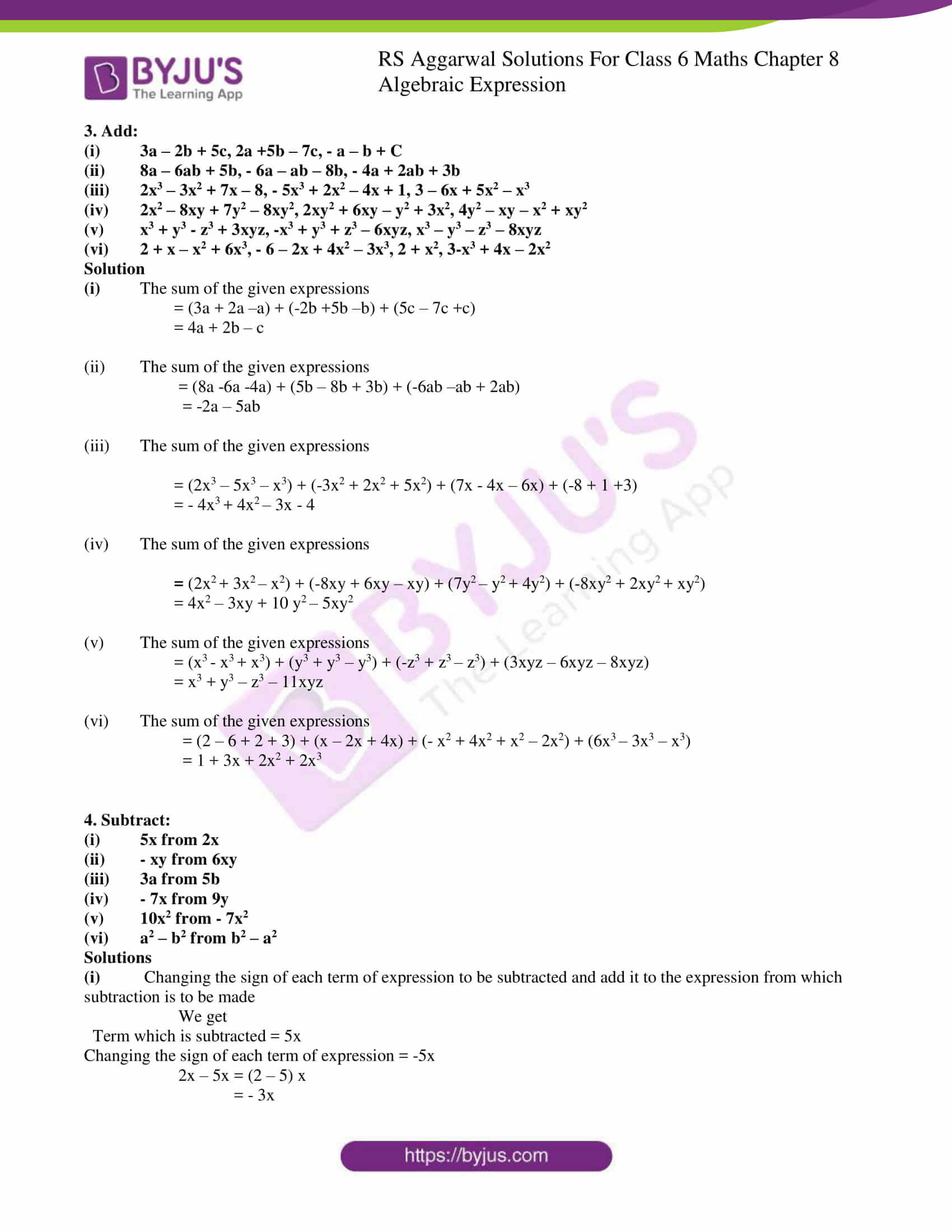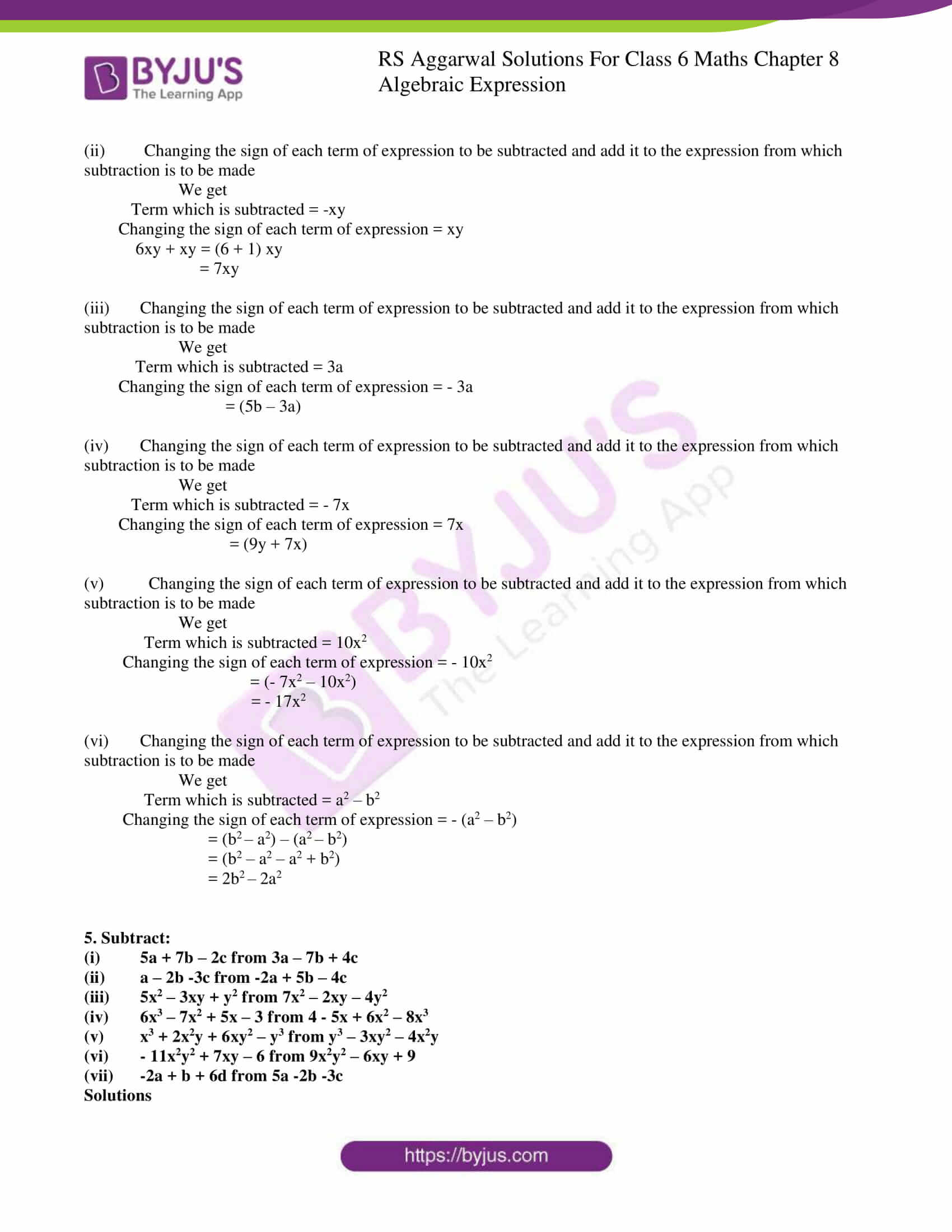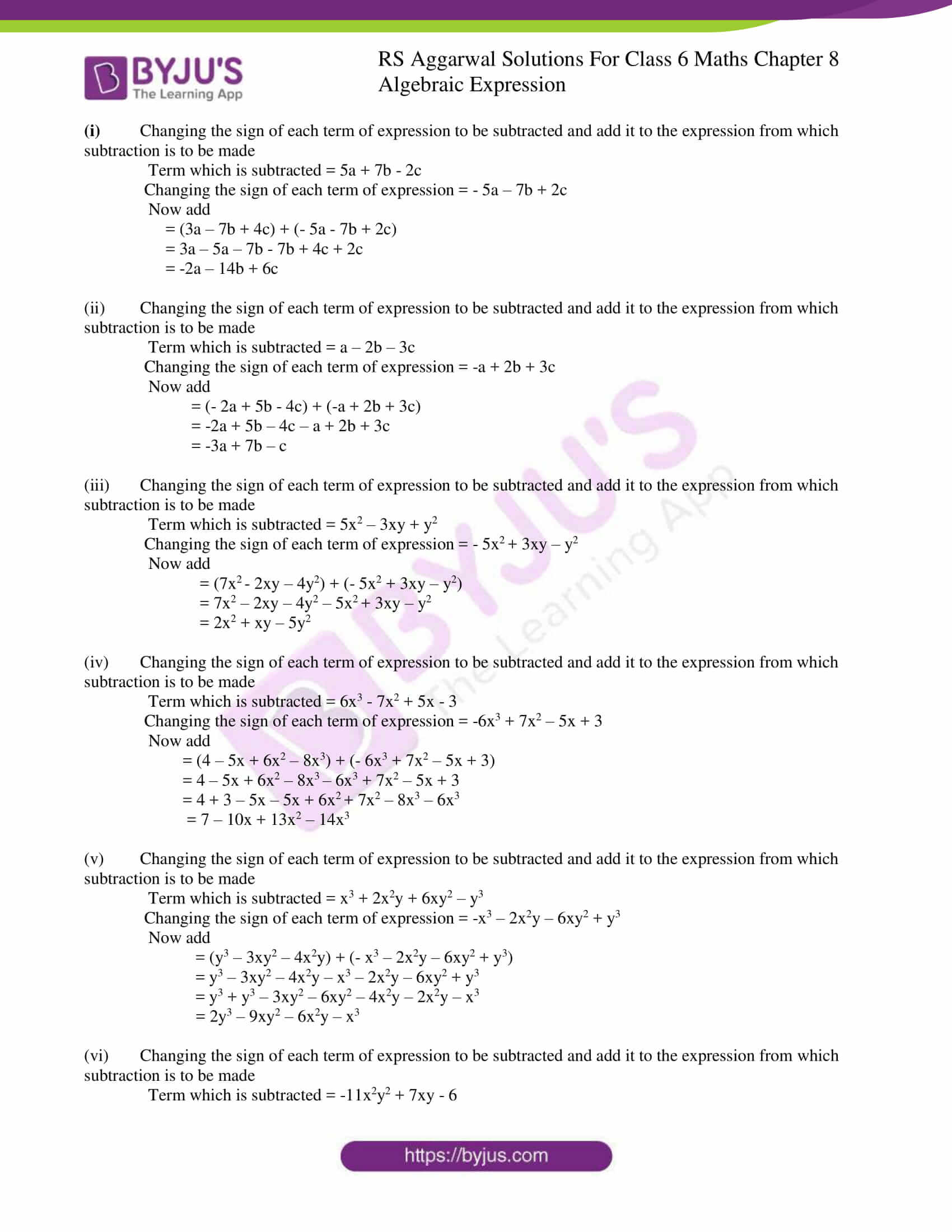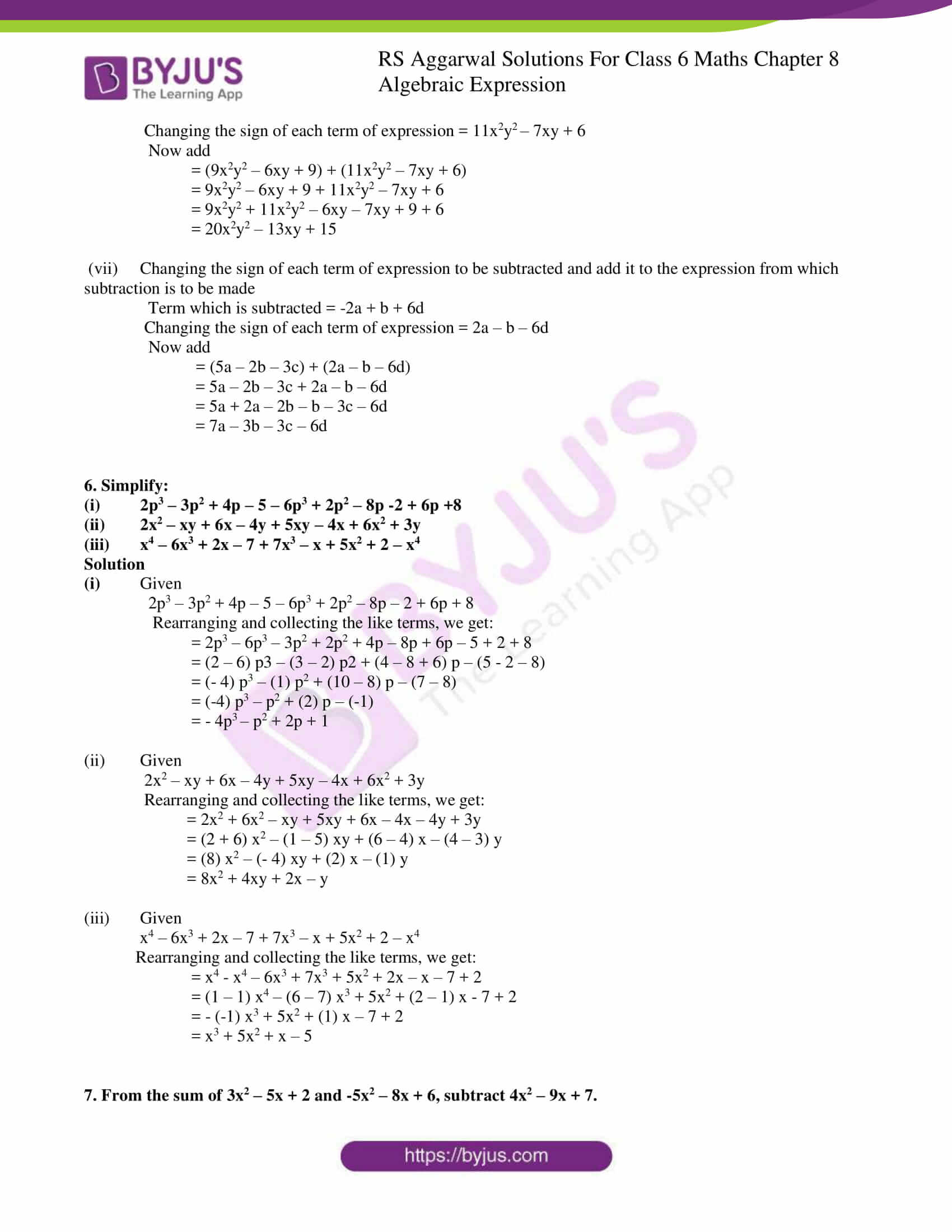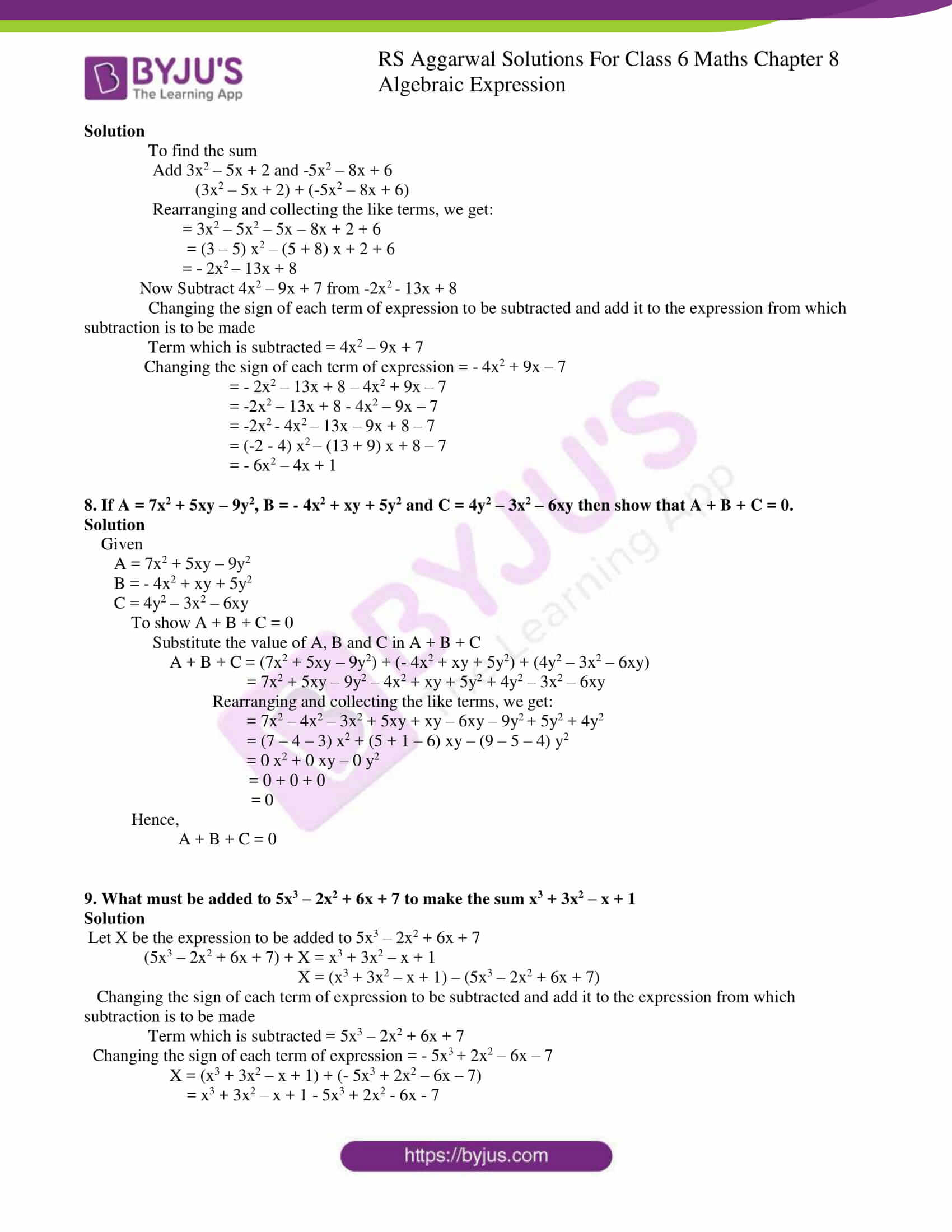### Access answers to Maths RS Aggarwal Solutions for Class 6 Chapter 8 Algebraic Expressions Exercise 8C

(i) 3x, 7x

(ii) 7y, -9y

(iii) 2xy, 5xy, -xy

(iv) 3x, 2y

(v) 2x2, -3x2, 7x2

(vi) 7xyz, -5xyz, 9xyz, -8xyz

(vii) 6a3, -4a3, 10a3, -8a3

(viii) x2 – a2, – 5x2 + 2a2, -4x2 + 4a2

Solutions

(i) The required sum = 3x + 7x

= (3 + 7) x

= 10x

(ii) The required sum = 7y + (-9y)

= 7y – 9y

= (7 -9) y

= -2y

(iii) The required sum = 2xy + 5xy + (-xy)

= 2xy + 5xy – xy

= (2x + 5x –x) y

= 6xy

(iv) The required sum = 3x + 2y

= 3x + 2y

(v) The required sum = 2x2 + (-3x2) + 7x2

= 2x2 – 3x2 + 7x2

= (2 – 3 + 7) x2

= 6x2

(vi) The required sum = 7xyz + (-5xyz) + 9xyz + (-8xyz)

= 7xyz – 5xyz + 9xyz – 8xyz

= (7 – 5 + 9 – 8) xyz

= (16 – 13) xyz

= 3xyz

(vii) The required sum = 6a3 + (-4a3) + 10a3 + (-8a3)

= 6a3 – 4a3 + 10a3 – 8a3

= (6 – 4 + 10 – 8) a3

= (16 – 12) a3

= 4a3

(viii) The required sum = (x2 – a2) + (-5x2 + 2a2) + (-4x2 + 4a2)

= x2– a2 – 5x2 + 2a2 – 4x2 + 4a2

= (1 – 5 – 4) x2 – (1 – 2 – 4) a2

= (1 – 9) x2 – (1 – 6) a2

= -8x2 + 5a2

(i) x – 3y – 2z (ii) m2 – 4m + 5 (iii) 2x2 – 3xy + y2

5x + 7y – z -2m2 + 6m – 6 – 7x2 – 5xy – 2y2

-7x – 2y + 4z -m2 – 2m – 7 4x2 + xy – 6y2

__________ __________ ____________

__________ __________ ____________

(iv) 4xy – 5yz – 7z

– 5xy +2yz + zx

– 2xy -3yz +3zx

____________

______________

Solutions

(i) x – 3y – 2z

5x + 7y – z

-7x – 2y + 4z

____________________

-x + 2y + z

____________________

(ii) m 2– 4m + 5

-2m2 + 6m – 6

– m2 – 2m – 7

_______________

-2m2 +0m – 8

= -2m2 – 8

________________

(iii) 2x2 – 3xy + y2

-7x2 – 5xy – 2y2

4x2 + xy – 6y2

___________________________

-x2 – 7xy – 7y2

_____________________________

(iv) 4xy – 5yz – 7zx

-5xy + 2yz + zx

-2xy – 3yz + 3zx

_________________

-3xy -6yz -3zx

________________

(i) 3a – 2b + 5c, 2a +5b – 7c, – a – b + C

(ii) 8a – 6ab + 5b, – 6a – ab – 8b, – 4a + 2ab + 3b

(iii) 2x3 – 3x2 + 7x – 8, – 5x3 + 2x2 – 4x + 1, 3 – 6x + 5x2 – x3

(iv) 2x2 – 8xy + 7y2 – 8xy2, 2xy2 + 6xy – y2 + 3x2, 4y2 – xy – x2 + xy2

(v) x3 + y3 – z3 + 3xyz, -x3 + y3 + z3 – 6xyz, x3 – y3 – z3 – 8xyz

(vi) 2 + x – x2 + 6x3, – 6 – 2x + 4x2 – 3x3, 2 + x2, 3-x3 + 4x – 2x2

Solution

(i) The sum of the given expressions

= (3a + 2a –a) + (-2b +5b –b) + (5c – 7c +c)

= 4a + 2b – c

(ii) The sum of the given expressions

= (8a -6a -4a) + (5b – 8b + 3b) + (-6ab –ab + 2ab)

= -2a – 5ab

(iii) The sum of the given expressions

= (2x3 – 5x3 – x3) + (-3x2 + 2x2 + 5x2) + (7x – 4x – 6x) + (-8 + 1 +3)

= – 4x3 + 4x2 – 3x – 4

(iv) The sum of the given expressions

= (2x2 + 3x2 – x2) + (-8xy + 6xy – xy) + (7y2 – y2 + 4y2) + (-8xy2 + 2xy2 + xy2)

= 4x2 – 3xy + 10 y2 – 5xy2

(v) The sum of the given expressions

= (x3 – x3 + x3) + (y3 + y3 – y3) + (-z3 + z3 – z3) + (3xyz – 6xyz – 8xyz)

= x3 + y3 – z3 – 11xyz

(vi) The sum of the given expressions

= (2 – 6 + 2 + 3) + (x – 2x + 4x) + (- x2 + 4x2 + x2 – 2x2) + (6x3 – 3x3 – x3)

= 1 + 3x + 2x2 + 2x3

4. Subtract:

(i) 5x from 2x

(ii) – xy from 6xy

(iii) 3a from 5b

(iv) – 7x from 9y

(v) 10x2 from – 7x2

(vi) a2 – b2 from b2 – a2

Solutions

(i) Changing the sign of each term of expression to be subtracted and add it to the expression from which subtraction is to be made

We get

Term which is subtracted = 5x

Changing the sign of each term of expression = -5x

2x – 5x = (2 – 5) x

= – 3x

(ii) Changing the sign of each term of expression to be subtracted and add it to the expression from which subtraction is to be made

We get

Term which is subtracted = -xy

Changing the sign of each term of expression = xy

6xy + xy = (6 + 1) xy

= 7xy

(iii) Changing the sign of each term of expression to be subtracted and add it to the expression from which subtraction is to be made

We get

Term which is subtracted = 3a

Changing the sign of each term of expression = – 3a

= (5b – 3a)

(iv) Changing the sign of each term of expression to be subtracted and add it to the expression from which subtraction is to be made

We get

Term which is subtracted = – 7x

Changing the sign of each term of expression = 7x

= (9y + 7x)

(v) Changing the sign of each term of expression to be subtracted and add it to the expression from which subtraction is to be made

We get

Term which is subtracted = 10x2

Changing the sign of each term of expression = – 10x2

= (- 7x2 – 10x2)

= – 17x2

(vi) Changing the sign of each term of expression to be subtracted and add it to the expression from which subtraction is to be made

We get

Term which is subtracted = a2 – b2

Changing the sign of each term of expression = – (a2 – b2)

= (b2 – a2) – (a2 – b2)

= (b2 – a2 – a2 + b2)

= 2b2 – 2a2

5. Subtract:

(i) 5a + 7b – 2c from 3a – 7b + 4c

(ii) a – 2b -3c from -2a + 5b – 4c

(iii) 5x2 – 3xy + y2 from 7x2 – 2xy – 4y2

(iv) 6x3 – 7x2 + 5x – 3 from 4 – 5x + 6x2 – 8x3

(v) x3 + 2x2y + 6xy2 – y3 from y3 – 3xy2 – 4x2y

(vi) – 11x2y2 + 7xy – 6 from 9x2y2 – 6xy + 9

(vii) -2a + b + 6d from 5a -2b -3c

Solutions

(i) Changing the sign of each term of expression to be subtracted and add it to the expression from which subtraction is to be made

Term which is subtracted = 5a + 7b – 2c

Changing the sign of each term of expression = – 5a – 7b + 2c

= (3a – 7b + 4c) + (- 5a – 7b + 2c)

= 3a – 5a – 7b – 7b + 4c + 2c

= -2a – 14b + 6c

(ii) Changing the sign of each term of expression to be subtracted and add it to the expression from which subtraction is to be made

Term which is subtracted = a – 2b – 3c

Changing the sign of each term of expression = -a + 2b + 3c

= (- 2a + 5b – 4c) + (-a + 2b + 3c)

= -2a + 5b – 4c – a + 2b + 3c

= -3a + 7b – c

(iii) Changing the sign of each term of expression to be subtracted and add it to the expression from which subtraction is to be made

Term which is subtracted = 5x2 – 3xy + y2

Changing the sign of each term of expression = – 5x2 + 3xy – y2

= (7x2 – 2xy – 4y2) + (- 5x2 + 3xy – y2)

= 7x2 – 2xy – 4y2 – 5x2 + 3xy – y2

= 2x2 + xy – 5y2

(iv) Changing the sign of each term of expression to be subtracted and add it to the expression from which subtraction is to be made

Term which is subtracted = 6x3 – 7x2 + 5x – 3

Changing the sign of each term of expression = -6x3 + 7x2 – 5x + 3

= (4 – 5x + 6x2 – 8x3) + (- 6x3 + 7x2 – 5x + 3)

= 4 – 5x + 6x2 – 8x3 – 6x3 + 7x2 – 5x + 3

= 4 + 3 – 5x – 5x + 6x2 + 7x2 – 8x3 – 6x3

= 7 – 10x + 13x2 – 14x3

(v) Changing the sign of each term of expression to be subtracted and add it to the expression from which subtraction is to be made

Term which is subtracted = x3 + 2x2y + 6xy2 – y3

Changing the sign of each term of expression = -x3 – 2x2y – 6xy2 + y3

= (y3 – 3xy2 – 4x2y) + (- x3 – 2x2y – 6xy2 + y3)

= y3 – 3xy2 – 4x2y – x3 – 2x2y – 6xy2 + y3

= y3 + y3 – 3xy2 – 6xy2 – 4x2y – 2x2y – x3

= 2y3 – 9xy2 – 6x2y – x3

(vi) Changing the sign of each term of expression to be subtracted and add it to the expression from which subtraction is to be made

Term which is subtracted = -11x2y2 + 7xy – 6

Changing the sign of each term of expression = 11x2y2 – 7xy + 6

= (9x2y2 – 6xy + 9) + (11x2y2 – 7xy + 6)

= 9x2y2 – 6xy + 9 + 11x2y2 – 7xy + 6

= 9x2y2 + 11x2y2 – 6xy – 7xy + 9 + 6

= 20x2y2 – 13xy + 15

(vii) Changing the sign of each term of expression to be subtracted and add it to the expression from which subtraction is to be made

Term which is subtracted = -2a + b + 6d

Changing the sign of each term of expression = 2a – b – 6d

= (5a – 2b – 3c) + (2a – b – 6d)

= 5a – 2b – 3c + 2a – b – 6d

= 5a + 2a – 2b – b – 3c – 6d

= 7a – 3b – 3c – 6d

6. Simplify:

(i) 2p3 – 3p2 + 4p – 5 – 6p3 + 2p2 – 8p -2 + 6p +8

(ii) 2x2 – xy + 6x – 4y + 5xy – 4x + 6x2 + 3y

(iii) x4 – 6x3 + 2x – 7 + 7x3 – x + 5x2 + 2 – x4

Solution

(i) Given

2p3 – 3p2 + 4p – 5 – 6p3 + 2p2 – 8p – 2 + 6p + 8

Rearranging and collecting the like terms, we get:

= 2p3 – 6p3 – 3p2 + 2p2 + 4p – 8p + 6p – 5 + 2 + 8

= (2 – 6) p3 – (3 – 2) p2 + (4 – 8 + 6) p – (5 – 2 – 8)

= (- 4) p3 – (1) p2 + (10 – 8) p – (7 – 8)

= (-4) p3 – p2 + (2) p – (-1)

= – 4p3 – p2 + 2p + 1

(ii) Given

2x2 – xy + 6x – 4y + 5xy – 4x + 6x2 + 3y

Rearranging and collecting the like terms, we get:

= 2x2 + 6x2 – xy + 5xy + 6x – 4x – 4y + 3y

= (2 + 6) x2 – (1 – 5) xy + (6 – 4) x – (4 – 3) y

= (8) x2 – (- 4) xy + (2) x – (1) y

= 8x2 + 4xy + 2x – y

(iii) Given

x4 – 6x3 + 2x – 7 + 7x3 – x + 5x2 + 2 – x4

Rearranging and collecting the like terms, we get:

= x4 – x4 – 6x3 + 7x3 + 5x2 + 2x – x – 7 + 2

= (1 – 1) x4 – (6 – 7) x3 + 5x2 + (2 – 1) x – 7 + 2

= – (-1) x3 + 5x2 + (1) x – 7 + 2

= x3 + 5x2 + x – 5

7. From the sum of 3x2 – 5x + 2 and -5x2 – 8x + 6, subtract 4x2 – 9x + 7.

Solution

To find the sum

Add 3x2 – 5x + 2 and -5x2 – 8x + 6

(3x2 – 5x + 2) + (-5x2 – 8x + 6)

Rearranging and collecting the like terms, we get:

= 3x2 – 5x2 – 5x – 8x + 2 + 6

= (3 – 5) x2 – (5 + 8) x + 2 + 6

= – 2x2 – 13x + 8

Now Subtract 4x2 – 9x + 7 from -2x2 – 13x + 8

Changing the sign of each term of expression to be subtracted and add it to the expression from which subtraction is to be made

Term which is subtracted = 4x2 – 9x + 7

Changing the sign of each term of expression = – 4x2 + 9x – 7

= – 2x2 – 13x + 8 – 4x2 + 9x – 7

= -2x2 – 13x + 8 – 4x2 – 9x – 7

= -2x2 – 4x2 – 13x – 9x + 8 – 7

= (-2 – 4) x2 – (13 + 9) x + 8 – 7

= – 6x2 – 4x + 1

8. If A = 7x2 + 5xy – 9y2, B = – 4x2 + xy + 5y2 and C = 4y2 – 3x2 – 6xy then show that A + B + C = 0.

Solution

Given

A = 7x2 + 5xy – 9y2

B = – 4x2 + xy + 5y2

C = 4y2 – 3x2 – 6xy

To show A + B + C = 0

Substitute the value of A, B and C in A + B + C

A + B + C = (7x2 + 5xy – 9y2) + (- 4x2 + xy + 5y2) + (4y2 – 3x2 – 6xy)

= 7x2 + 5xy – 9y2 – 4x2 + xy + 5y2 + 4y2 – 3x2 – 6xy

Rearranging and collecting the like terms, we get:

= 7x2 – 4x2 – 3x2 + 5xy + xy – 6xy – 9y2 + 5y2 + 4y2

= (7 – 4 – 3) x2 + (5 + 1 – 6) xy – (9 – 5 – 4) y2

= 0 x2 + 0 xy – 0 y2

= 0 + 0 + 0

= 0

Hence,

A + B + C = 0

9. What must be added to 5x3 – 2x2 + 6x + 7 to make the sum x3 + 3x2 – x + 1

Solution

Let X be the expression to be added to 5x3 – 2x2 + 6x + 7

(5x3 – 2x2 + 6x + 7) + X = x3 + 3x2 – x + 1

X = (x3 + 3x2 – x + 1) – (5x3 – 2x2 + 6x + 7)

Changing the sign of each term of expression to be subtracted and add it to the expression from which subtraction is to be made

Term which is subtracted = 5x3 – 2x2 + 6x + 7

Changing the sign of each term of expression = – 5x3 + 2x2 – 6x – 7

X = (x3 + 3x2 – x + 1) + (- 5x3 + 2x2 – 6x – 7)

= x3 + 3x2 – x + 1 – 5x3 + 2x2 – 6x – 7

Rearranging and collecting the like terms, we get:

= x3 – 5x3 + 3x2 + 2x2 – x – 6x + 1 – 7

= (1 – 5) x3 + (3 + 2) x2 – (1 + 6) x + 1 – 7

= – 4 x3 + 5 x2 + 7 x – 6

∴ – 4 x3 + 5 x2 + 7 x – 6 must be added to 5x3 – 2x2 + 6x + 7 to make the sum x3 + 3x2 – x + 1